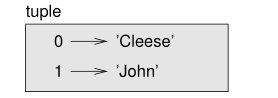# # 第十二章 元组

## # 12.1 元组不可修改

``````    >>> t = 'a', 'b', 'c', 'd', 'e'

``````
1
2

``````
>>> t = ('a', 'b', 'c', 'd', 'e')

``````
1
2
3

``````    >>> t1 = 'a',
>>> type(t1)
<class 'tuple'>

``````
1
2
3
4

``````
>>> t2 = ('a')
>>> type(t2)
<class 'str'>

``````
1
2
3
4
5

``````    >>> t = tuple()
>>> t
()

``````
1
2
3
4

``````
>>> t = tuple('lupins')
>>> t
('l', 'u', 'p', 'i', 'n', 's')

``````
1
2
3
4
5

tuple 是内置函数命了，所以你就不能用来作为变量名了。

``````    >>> t = ('a', 'b', 'c', 'd', 'e')
>>> t
'a'
``````
1
2
3

``````    >>> t[1:3]
('b', 'c')
``````
1
2

``````
>>> t = 'A'
TypeError: object doesn't support item assignment

``````
1
2
3
4

``````    >>> t = ('A',) + t[1:]
>>> t
('A', 'b', 'c', 'd', 'e')

``````
1
2
3
4

``````    >>> (0, 1, 2) < (0, 3, 4)
True
>>> (0, 1, 2000000) < (0, 3, 4)
True
``````
1
2
3
4

## # 12.2 元组赋值

``````
>>> temp = a
>>> a = b
>>> b = temp

``````
1
2
3
4
5

``````    >>> a, b = b, a
``````
1

``````

>>> a, b = 1, 2, 3
ValueError: too many values to unpack
``````
1
2
3
4

``````
``````
1
2
3

split 的返回值是一个有两个元素的列表；第一个元素赋值给了 uname 这个变量，第二个赋值给了 domain 这个变量。

``````
>>> uname
'monty'
>>> domain
'python.org'

``````
1
2
3
4
5
6

## # 12.3 用元组做返回值

``````
>>> t = divmod(7, 3)
>>> t
(2, 1)

``````
1
2
3
4
5

``````    >>> quot, rem = divmod(7, 3)
>>> quot
2
>>> rem
1

``````
1
2
3
4
5
6

``````    def min_max(t):
return min(t), max(t)

``````
1
2
3

max 和 min 都是内置函数，会找到序列中的最大值或者最小值，min_max 这个函数会同时求得最大值和最小值，然后把这两个值作为元组来返回。

## # 12.4 参数长度可变的元组

``````
def printall(*args):
print(args)

``````
1
2
3
4

``````
>>> printall(1, 2.0, '3')
(1, 2.0, '3')

``````
1
2
3
4

``````
>>> t = (7, 3)
>>> divmod(t)
TypeError: divmod expected 2 arguments, got 1

``````
1
2
3
4
5

``````    >>> divmod(*t)
(2, 1)
``````
1
2

``````

>>> max(1, 2, 3)
3

``````
1
2
3
4
5

``````
>>> sum(1, 2, 3)
TypeError: sum expected at most 2 arguments, got 3

``````
1
2
3
4

## # 12.5 列表和元组

zip 是一个内置函数，接收两个或更多的序列作为参数，然后返回返回一个元组列表，该列表中每个元组都包含了从各个序列中的一个元素。这个函数名的意思就是拉锁，就是把不相关的两排拉锁齿连接到一起。

``````
>>> s = 'abc'
>>> t = [0, 1, 2]
>>> zip(s, t)
<zip object at 0x7f7d0a9e7c48>

``````
1
2
3
4
5
6

``````
>>> for pair in zip(s, t): ...
print(pair) ...
('a', 0) ('b', 1) ('c', 2)

``````
1
2
3
4
5

zip 对象是一种迭代器，也就是某种可以迭代整个序列的对象。迭代器和列表有些相似，但不同于列表的是，你无法通过索引来选择迭代器中的指定元素。

``````

>>> list(zip(s, t))
[('a', 0), ('b', 1), ('c', 2)]

``````
1
2
3
4
5

``````

>>> list(zip('Anne', 'Elk'))
[('A', 'E'), ('n', 'l'), ('n', 'k')]

``````
1
2
3
4
5

``````    t = [('a', 0), ('b', 1), ('c', 2)]
for letter, number in t:
print(number, letter)

``````
1
2
3
4

``````    0 a 1 b 2 c
``````
1

``````    def has_match(t1, t2):
for x, y in zip(t1, t2):
if x == y:
return True
return False
``````
1
2
3
4
5

``````    for index, element in enumerate('abc'):
print(index, element)

``````
1
2
3

enumerate 函数的返回值是一个枚举对象，它会遍历整个成对序列；每一对都包括一个索引（从0开始）以及给定序列的一个元素。在本节的例子中，输出依然如下：

``````
0 a 1 b 2 c
``````
1
2

## # 12.6 词典与元组

``````
>>> d = {'a':0, 'b':1, 'c':2}
>>> t = d.items()
>>> t
dict_items([('c', 2), ('a', 0), ('b', 1)])
``````
1
2
3
4
5

``````
>>> for key, value in d.items():
...     print(key, value)
... c 2 a 0 b 1
``````
1
2
3
4

``````    >>> t = [('a', 0), ('c', 2), ('b', 1)]
>>> d = dict(t)
>>> d
{'a': 0, 'c': 2, 'b': 1}
``````
1
2
3
4

``````
>>> d = dict(zip('abc', range(3)))
>>> d
{'a': 0, 'c': 2, 'b': 1}
``````
1
2
3
4

``````    directory[last, first] = number
``````
1

``````

for last, first in directory:
print(first, last, directory[last,first])
``````
1
2
3
4Figure 12.1: State diagram.Figure 12.2: State diagram.

## # 12.8 调试

``````
>>> from structshape import structshape
>>> t = [1, 2, 3]
>>> structshape(t)
'list of 3 int'
``````
1
2
3
4
5

``````
>>> t2 = [[1,2], [3,4], [5,6]]
>>> structshape(t2)
'list of 3 list of 2 int'
``````
1
2
3
4

``````
>>> t3 = [1, 2, 3, 4.0, '5', '6', , , 9]
>>> structshape(t3)
'list of (3 int, float, 2 str, 2 list of int, int)'
``````
1
2
3
4

``````    >>> s = 'abc'
>>> lt = list(zip(t, s))
>>> structshape(lt)
'list of 3 tuple of (int, str)'
``````
1
2
3
4

``````
>>> d = dict(lt)
>>> structshape(d)
'dict of 3 int->str'
``````
1
2
3
4

## # 12.9 Glossary 术语列表

tuple: An immutable sequence of elements.

tuple assignment: An assignment with a sequence on the right side and a tuple of variables on the left. The right side is evaluated and then its elements are assigned to the variables on the left.

gather: The operation of assembling a variable-length argument tuple.

scatter: The operation of treating a sequence as a list of arguments.

zip object: The result of calling a built-in function zip; an object that iterates through a sequence of tuples.

iterator: An object that can iterate through a sequence, but which does not provide list operators and methods.

data structure: A collection of related values, often organized in lists, dictionaries, tuples, etc.

shape error: An error caused because a value has the wrong shape; that is, the wrong type or size.

## # 12.10 练习

### # 练习2

1. 写一个函数，读取一个文件中的一个单词列表（参考9.1），然后输出所有的变位词。

['deltas', 'desalt', 'lasted', 'salted', 'slated', 'staled'] ['retainers', 'ternaries'] ['generating', 'greatening'] ['resmelts', 'smelters', 'termless']

2.修改一下之前的程序，让它先输出变位词列表中最长的，然后是其次长的，依此类推。

1. 在拼字游戏中，当你已经有七个字母的时候，再添加一个字母就能组成一个八个字母的单词，这就 TMD『bingo』 了（什么鬼东西？老外拼字游戏就跟狗一样，翻着恶心死了）。然后哪八个字母组合起来最可能得到 bingo？提示：有七个。（简直就是狗一样的题目，麻烦死了，这里数据结构大家学会了就好了。）样例代码.

### # 练习4

1. 你也许需要写一个函数，接收一个单词然后计算一下这个单词去掉一个字母能得到单词组成的列表。列表中这些单词就如同原始单词的孩子一样。

2. 只要一个 单词的孩子还可以缩减，那这个单词本身就亏缩减。你可以认为空字符串是可以缩减的，这样来作为一个基准条件。

3. 我上面提供的 words.txt 这个词表，不包含单个字母的单词。所以你需要自行添加 I、a 以及空字符串上去。

4. 要提高程序性能的话，你最好存储住已经算出来能被继续缩减的单词。样例代码